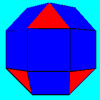#### You may also like### Eight Dominoes

Using the 8 dominoes make a square where each of the columns and rows adds up to 8### Rhombicubocts

Each of these solids is made up with 3 squares and a triangle around each vertex. Each has a total of 18 square faces and 8 faces that are equilateral triangles. How many faces, edges and vertices does each solid have?### Prime Magic

Place the numbers 1, 2, 3,..., 9 one on each square of a 3 by 3 grid so that all the rows and columns add up to a prime number. How many different solutions can you find?

# Notes on a Triangle

##### Age 11 to 14 Challenge Level:

You can pause the film at any point to look carefully at the images.